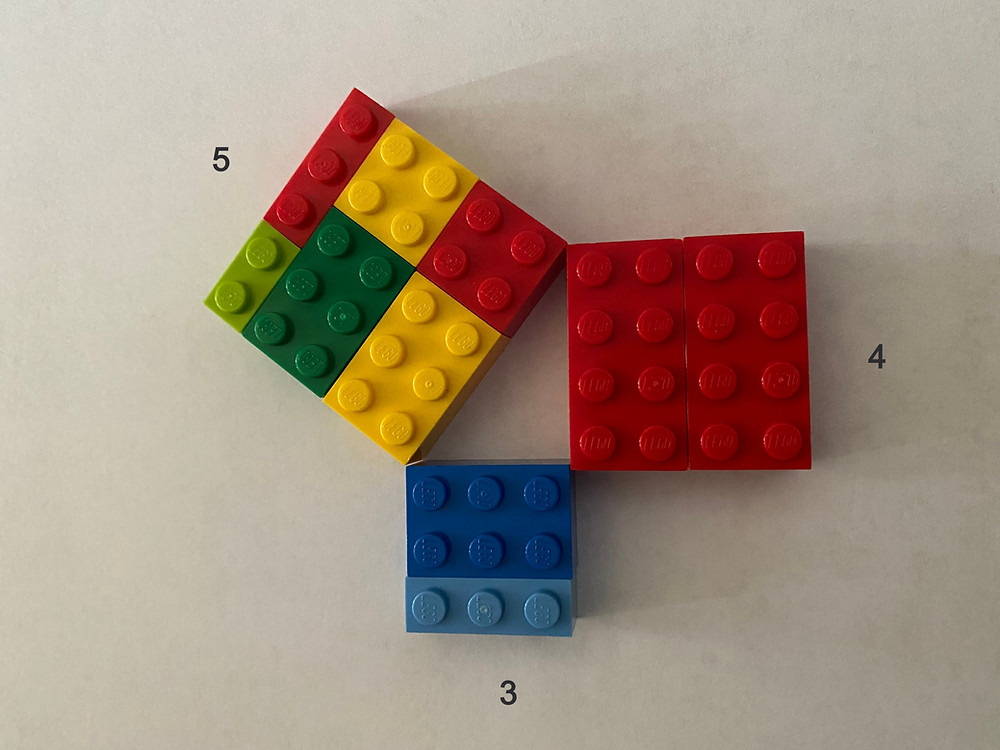Search
•Friendly Blogger

# Mystery of Pythagoras theorem-Facts and Proof

Χαίρετε! Welcome to Greece! Have you explored the wondrous beauty this place holds? It's stunning! Oh, by the way, I found an amazing concept which I must share with you. While we are at it, let's visit the place where this brilliant mathematician was born. Let’s set forth to Samos Ionia as we walk to breathe in the great nature around us. και πάμε. You might have guessed its greek philosopher and mathematician Pythagoras (570 to ca. 490 BCE) and eponym founder for the Pythagorean theorem. What is the mystery of Pythagoras theorem - Facts and Proof?

# How do Pythagoras theorem work and its Applications?

Pythagoras theorem is the most researched and has 350 ways to prove the fabulous theorem. Before we get started let's find how this theorem works. For a right-angle triangle, the sum of the square of side a and the square of side b is equal to the square on the hypotenuse which is side c.### Figure 1Pythagoras theorem has life applications in most structural buildings, architecture, astronomy (to calculate the path of the rocket, planet orbit, and distance). For example, in the picture below a person drone hovers above the ground at a distance. We can calculate the height of the drone from the ground using the Pythagoras theorem.## How to prove the Pythagorean theorem?

Before we begin, let’s clarify how many ways are there to prove this stunning Pythagorean theorem. This question was lurking in my head and I knew it must be answered. Now to get to the proof! Heads up, there are multiple ways to prove the Pythagorean Theorem, but here we will be leaning and looking over just a few. Got all that. Great, let’s get started.

### 1. Pythagorean theorem -Proof by rearrangement

This method was used by Pythagoras himself to prove the theorem. We will need 4 identical right-angle triangles. First, we will need to arrange the right-angle triangles, so the hypotenuses form a tilted or turned square. Now, the area of that square is c^2. Next, you will need to arrange the triangle into two rectangles. This will make them depart and leave smaller squares on each side. Those squares are a^2 and b^2

To our surprise, the sum of the area of a^2+b^2=c^2 small squares (figure 2) is equal to the area of big square c^2 which is formed by connecting all the hypotenuses! Now to get to the facts and explain how this astounding method works. The total area of the shape or figure did not change, and the areas of the right-angle triangles remained the same. Therefore, the big empty square which we labelled c^2 is equal to the sum of the two smaller squares a^2 and b^2. By using this method, we again stumbled upon the famous equation### Proof by rearrangement (Figure 2)### 2. Pythagoras theorem - Splitting Triangles

Splitting triangle proof was elucidated by the Greek mathematician and Father of Geometry Elucid (mid 4th BC - mid 3rd BC). This method splits a right angle triangle into two smaller right-angled triangles. This uses the tenet that if the subsequent angles of the two triangles are the same the ratio of their sides is the same too (figure 3). For the three right-angled triangles, there is an expression you can write for there sides.

AC/CD = BC/AC & AB/BD = BC/AB.

After you write this expression you will need to rearrange the terms.

AC^2 = BC * CD & AB^2 = BC * BD.

Next, you will have to add the two equations and then simplify to get the outcome as:

AB^2+AC^2=BC^2 or in other words a^2+b^2=c^2

Did you catch all proof? That is a whole lot of equations.

### Proof by Splitting Triangles (Figure 3)### 3. Turning Method to prove Pythagoras Theorem

Not convinced yet, try this trick and we are sure that you will believe in this amazing theorem. On a turntable connect three square boxes of the same or equal depth to each other. This will form a right-angled triangle in the center. Then, fill the largest square with beads, then we turn the turntable you will notice that the beads will exactly fill the other two smaller squares and this happens vice versa (figure 1).

Now the finale- Pythagorean Theorem has many proofs. More than a hundred! I bet you can even add your own to this jumble of tricky geometry. Wow, we are here at Samos Ionia. Hope to see you next time! Bye! Oh, yeah, don’t forget to taste the Greek cuisine here it's delicious!